# QlikView App Dev

Discussion Board for collaboration related to QlikView App Development.

Announcements
Action-Packed Learning Awaits! QlikWorld 2023. April 17 - 20 in Las Vegas: REGISTER NOW
cancel
Showing results for
Did you mean: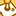Contributor

## Need help for using Rank

Can anyone tell me what's wrong with the following coding:

=Num(COUNT(DISTINCT{<CONTRACT_NUMBER= {"=Rank(COUNT(DISTINCT PO.PROC_AGENCY={'Supply Logistics APS'}),4)<=vTopN"}>}PO.PROC_AGENCY),'#,##0')

Actually, I want to use 02 conditions in this formula

1. Having procurement agency "Supply Logistics APS"

2. Apply to Top N records i.e.; Top 5, Top 10  or All Data

Thanks

Labels (1)
• ### Ranking on one dimension & one variable

6 RepliesMVP

One quickly spotted syntax issue.

=Num(COUNT(DISTINCT{<CONTRACT_NUMBER= {"=Rank(COUNT(DISTINCT {<PO.PROC_AGENCY={'Supply Logistics APS'}>}),4)<=vTopN"}>}PO.PROC_AGENCY),'#,##0')

If this doesn't resolve the issue, try to share a sample app explaining expected output in that context.Contributor
Author

My code is working fine, if I do not add the PO.Proc_agency condition, but in the formula I have to count number of contracts related with APS or ANS besides Top N records, so it means that now I have to cater 02 conditions in this formula.

I have changed my code as provided by you but nothing new happened as the result is 0 which is incorrect.MVP

I am very bad in helping without sample app,specially when it is non-generic problem. Try and see if you could share a sample app or at least explain with a sample data set.Employee

The following may be of some help, not sure:

https://community.qlik.com/t5/Qlik-Design-Blog/Recipe-for-a-Pareto-Analysis/ba-p/1468497

https://community.qlik.com/t5/Qlik-Design-Blog/Recipe-for-a-Pareto-Analysis-Revisited/ba-p/1473684

https://community.qlik.com/t5/Qlik-Design-Blog/Rank-Function/ba-p/1466430

Sorry I do not have anything better, but hopefully one of these may get you what you need.

Regards,
Brett

To help users find verified answers, please do not forget to use the "Accept as Solution" button on any post(s) that helped you resolve your problem or question.
I now work a compressed schedule, Tuesday, Wednesday and Thursday, so those will be the days I will reply to any follow-up posts.Contributor
Author

I hope that the following information will help you understand my issue.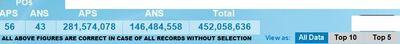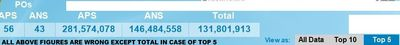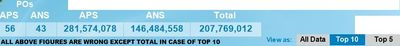The expression used against each 5 columns is given below:

EXPRESSION BEHIND 1ST COLUMN
=Count(DISTINCT{<PO.PROC_AGENCY={'Supply Logistics
APS'}>} PO_NUMBER)

EXPRESSION BEHIND 2ND COLUMN
=Count(DISTINCT{<PO.PROC_AGENCY={'Supply Logistics
ANS'}>} PO_NUMBER)

EXPRESSION BEHIND 3RD COLUMN
=Num(Sum(TOTAL{<PO.PROC_AGENCY={'Supply Logistics APS'}
>} PO.PO_AMOUNT+PO.GST_AMOUNT),'#,##0')

EXPRESSION BEHIND 4TH COLUMN
=Num(Sum(TOTAL{<PO.PROC_AGENCY={'Supply Logistics ANS'}
>} PO.PO_AMOUNT+PO.GST_AMOUNT),'#,##0')

EXPRESSION BEHIND 5TH COLUMN
=Num(SUM({<PO_NUMBER= {"=Rank(SUM(PO.PO_AMOUNT
+PO.GST_AMOUNT),4)<=vTopN"}>}PO.PO_AMOUNT
+PO.GST_AMOUNT),'#,##0')Contributor
Author

I hope that the following information will help you understand my issue.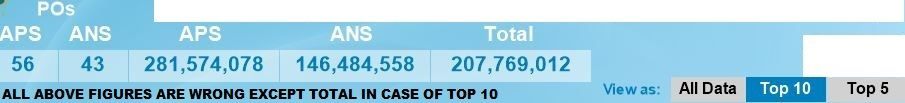The expression used against each 5 columns is given below:

EXPRESSION BEHIND 1ST COLUMN =Count(DISTINCT{<PO.PROC_AGENCY={'Supply Logistics APS'}>} PO_NUMBER) EXPRESSION BEHIND 2ND COLUMN =Count(DISTINCT{<PO.PROC_AGENCY={'Supply Logistics ANS'}>} PO_NUMBER) EXPRESSION BEHIND 3RD COLUMN =Num(Sum(TOTAL{<PO.PROC_AGENCY={'Supply Logistics APS'} >} PO.PO_AMOUNT+PO.GST_AMOUNT),'#,##0')

EXPRESSION BEHIND 4TH COLUMN =Num(Sum(TOTAL{<PO.PROC_AGENCY={'Supply Logistics ANS'} >} PO.PO_AMOUNT+PO.GST_AMOUNT),'#,##0')

EXPRESSION BEHIND 5TH COLUMN =Num(SUM({<PO_NUMBER= {"=Rank(SUM(PO.PO_AMOUNT +PO.GST_AMOUNT),4)<=vTopN"}>}PO.PO_AMOUNT +PO.GST_AMOUNT),'#,##0')Community Browser